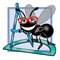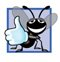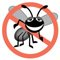# Formulating Algorithms: Counter-Controlled Repetition

### Formulating Algorithms Counter Controlled Repetition

To illustrate how algorithms are developed, we modify the GradeBook class of Chapter 3 to solve two variations of a problem that averages student grades. Consider the following problem statement:

A class of ten students took a quiz. The grades (integers in the range 0 to 100) for this quiz are available to you. Determine the class average on the quiz.

The class average is equal to the sum of the grades divided by the number of students. The algorithm for solving this problem on a computer must input each grade, keep track of the total of all grades input, perform the averaging calculation and print the result.

Pseudocode Algorithm with Counter-Controlled Repetition

Let's use pseudocode to list the actions to execute and specify the order in which they should execute. We use counter-controlled repetition to input the grades one at a time. This technique uses a variable called a counter (or control variable) to control the number of times a set of statements will execute. Counter-controlled repetition is often called definite repetition, because the number of repetitions is known before the loop begins executing. In this example, repetition terminates when the counter exceeds 10. This section presents a fully developed pseudocode algorithm (Fig. 4.5) and a version of class GradeBook (Fig. 4.6) that implements the algorithm in a Java method. The section then presents an application (Fig. 4.7) that demonstrates the algorithm in action. In Section 4.9, we demonstrate how to use pseudocode to develop such an algorithm from scratch.

Figure 4.5. Pseudocode algorithm that uses counter-controlled repetition to solve the class-average problem.

 1   Set total to zero  2   Set grade counter to one  3  4   While grade counter is less than or equal to ten  5       Prompt the user to enter the next grade  6       Input the next grade  7       Add the grade into the total  8       Add one to the grade counter  9 10   Set the class average to the total divided by ten 11   Print the class average

Figure 4.6. Counter-controlled repetition: Class-average problem.

(This item is displayed on pages 136 - 138 in the print version)

 ``` 1 // Fig. 4.6: GradeBook.java 2 // GradeBook class that solves class-average problem using 3 // counter-controlled repetition. 4 import java.util.Scanner; // program uses class Scanner 5 6 public class GradeBook 7 { 8 private String courseName; // name of course this GradeBook represents 9 10 // constructor initializes courseName 11 public GradeBook( String name ) 12 { 13 courseName = name; // initializes courseName 14 } // end constructor 15 16 // method to set the course name 17 public void setCourseName( String name ) 18 { 19 courseName = name; // store the course name 20 } // end method setCourseName 21 22 // method to retrieve the course name 23 public String getCourseName() 24 { 25 return courseName; 26 } // end method getCourseName 27 28 // display a welcome message to the GradeBook user 29 public void displayMessage() 30 { 31 // getCourseName gets the name of the course 32 System.out.printf( "Welcome to the grade book for %s! ", 33 getCourseName() ); 34 } // end method displayMessage 35 36 // determine class average based on 10 grades entered by user 37 public void determineClassAverage() 38 { 39 // create Scanner to obtain input from command window 40 Scanner input = new Scanner( System.in ); 41 42 int total; // sum of grades entered by user 43 int gradeCounter; // number of the grade to be entered next 44 int grade; // grade value entered by user 45 int average; // average of grades 46 47 // initialization phase 48 total = 0; // initialize total 49 gradeCounter = 1; // initialize loop counter 50 51 // processing phase 52 while ( gradeCounter <= 10 ) // loop 10 times 53 { 54 System.out.print( "Enter grade: " ); // prompt 55 grade = input.nextInt(); // input next grade 56 total = total + grade; // add grade to total 57 gradeCounter = gradeCounter + 1; // increment counter by 1 58 } // end while 59 60 // termination phase 61 average = total / 10; // integer division yields integer result 62 63 // display total and average of grades 64 System.out.printf( " Total of all 10 grades is %d ", total ); 65 System.out.printf( "Class average is %d ", average ); 66 } // end method determineClassAverage 67 68 } // end class GradeBook ```

Figure 4.7. GradeBookTest class creates an object of class GradeBook (Fig. 4.6) and invokes its determineClassAverage method.

(This item is displayed on pages 139 - 140 in the print version)

``` 1 // Fig. 4.7: GradeBookTest.java
2 // Create GradeBook object and invoke its determineClassAverage method.
3
5 {
6 public static void main( String args[] )
7 {
9 // pass course name to constructor
11 "CS101 Introduction to Java Programming" );
12
13 myGradeBook.displayMessage(); // display welcome message
15 } // end main
16
17 } // end class GradeBookTest
```

 ```Welcome to the grade book for CS101 Introduction to Java Programming! Enter grade: 67 Enter grade: 78 Enter grade: 89 Enter grade: 67 Enter grade: 87 Enter grade: 98 Enter grade: 93 Enter grade: 85 Enter grade: 82 Enter grade: 100 Total of all 10 grades is 846 Class average is 84 ```

Software Engineering Observation 4.1Experience has shown that the most difficult part of solving a problem on a computer is developing the algorithm for the solution. Once a correct algorithm has been specified, the process of producing a working Java program from the algorithm is normally straightforward.

Note the references in the algorithm of Fig. 4.5 to a total and a counter. A total is a variable used to accumulate the sum of several values. A counter is a variable used to countin this case, the grade counter indicates which of the 10 grades is about to be entered by the user. Variables used to store totals are normally initialized to zero before being used in a program.

Implementing Counter-Controlled Repetition in Class GradeBook

Class GradeBook (Fig. 4.6) contains a constructor (lines 1114) that assigns a value to the class's instance variable courseName (declared in line 8). Lines 1720, 2326 and 2934 declare methods setCourseName, getCourseName and displayMessage, respectively. Lines 3766 declare method determineClassAverage, which implements the class-averaging algorithm described by the pseudocode in Fig. 4.5.

Line 40 declares and initializes Scanner variable input, which is used to read values entered by the user. Lines 4245 declare local variables total, gradeCounter, grade and average to be of type int. Variable grade stores the user input.

Note that the declarations (in lines 4245) appear in the body of method determinesClassAverage. Recall that variables declared in a method body are local variables and can be used only from the line of their declaration in the method to the closing right brace (}) of the method declaration. A local variable's declaration must appear before the variable is used in that method. A local variable cannot be accessed outside the method in which it is declared.

In the versions of class GradeBook in this chapter, we simply read and process a set of grades. The averaging calculation is performed in method determineClassAverage using local variableswe do not preserve any information about student grades in instance variables of the class. In later versions of the class (in Chapter 7, Arrays), we maintain the grades in memory using an instance variable that refers to a data structure known as an array. This allows a GradeBook object to perform various calculations on the same set of grades without requiring the user to enter the grades multiple times.

Good Programming Practice 4.5Separate declarations from other statements in methods with a blank line for readability.

The assignments (in lines 4849) initialize total to 0 and gradeCounter to 1. Note that these initializations occur before the variables are used in calculations. Variables grade and average (for the user input and calculated average, respectively) need not be initialized heretheir values will be assigned as they are input or calculated later in the method.

Common Programming Error 4.4Using the value of a local variable before it is initialized results in a compilation error. All local variables must be initialized before their values are used in expressions.

Error-Prevention Tip 4.1Initialize each counter and total, either in its declaration or in an assignment statement. Totals are normally initialized to 0. Counters are normally initialized to 0 or 1, depending on how they are used (we will show examples of when to use 0 and when to use 1).

Line 52 indicates that the while statement should continue looping (also called iterating) as long as the value of gradeCounter is less than or equal to 10. While this condition remains true, the while statement repeatedly executes the statements between the braces that delimit its body (lines 5358).

Line 54 displays the prompt "Enter grade:" at the command line. Line 55 reads the grade entered by the user and assigns it to variable grade. Then line 56 adds the new grade entered by the user to the total and assigns the result to total, which replaces its previous value.

Line 57 adds 1 to gradeCounter to indicate that the program has processed a grade and is ready to input the next grade from the user. Incrementing gradeCounter eventually causes gradeCounter to exceed 10. At that point the while loop terminates because its condition (line 52) becomes false.

When the loop terminates, line 61 performs the averaging calculation and assigns its result to the variable average. Line 64 uses System.out's printf method to display the text "Total of all 10 grades is " followed by variable total's value. Line 65 then uses printf to display the text "Class average is " followed by variable average's value. Method determineClassAverage returns control to the calling method (i.e., main in GradeBookTest of Fig. 4.7) after reaching line 66.

Class GradeBookTest (Fig. 4.7) creates an object of class GradeBook (Fig. 4.6) and demonstrates its capabilities. Lines 1011 of Fig. 4.7 create a new GradeBook object and assign it to variable myGradeBook. The String in line 11 is passed to the GradeBook constructor (lines 1114 of Fig. 4.6). Line 13 calls myGradeBook's displayMessage method to display a welcome message to the user. Line 14 then calls myGradeBook's determineClassAverage method to allow the user to enter 10 grades, for which the method then calculates and prints the averagethe method performs the algorithm shown in Fig. 4.5.

Notes on Integer Division and Truncation

The averaging calculation performed by method determineClassAverage in response to the method call at line 14 in Fig. 4.7 produces an integer result. The program's output indicates that the sum of the grade values in the sample execution is 846, which, when divided by 10, should yield the floating-point number 84.6. However, the result of the calculation total / 10 (line 61 of Fig. 4.6) is the integer 84, because total and 10 are both integers. Dividing two integers results in integer divisionany fractional part of the calculation is lost (i.e., truncated). We will see how to obtain a floating-point result from the averaging calculation in the next section.

Common Programming Error 4.5Assuming that integer division rounds (rather than truncates) can lead to incorrect results. For example, 7 ÷ 4, which yields 1.75 in conventional arithmetic, truncates to 1 in integer arithmetic, rather than rounding to 2.Java How to Program (6th Edition) (How to Program (Deitel))
ISBN: 0131483986
EAN: 2147483647
Year: 2003
Pages: 615